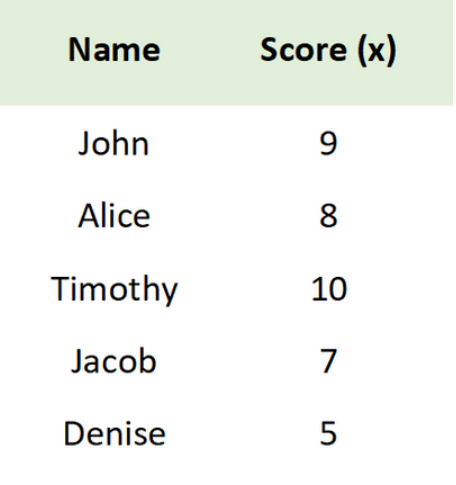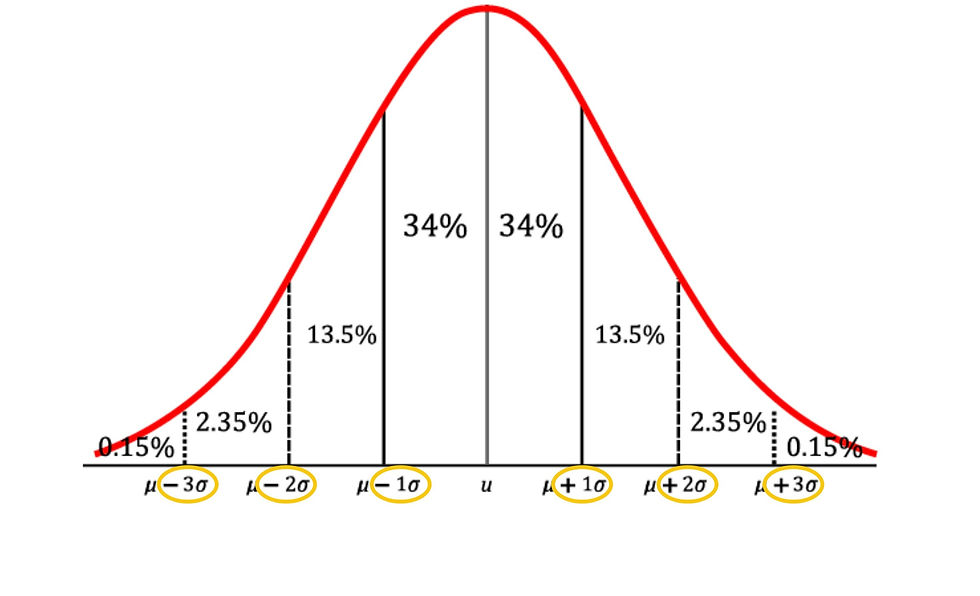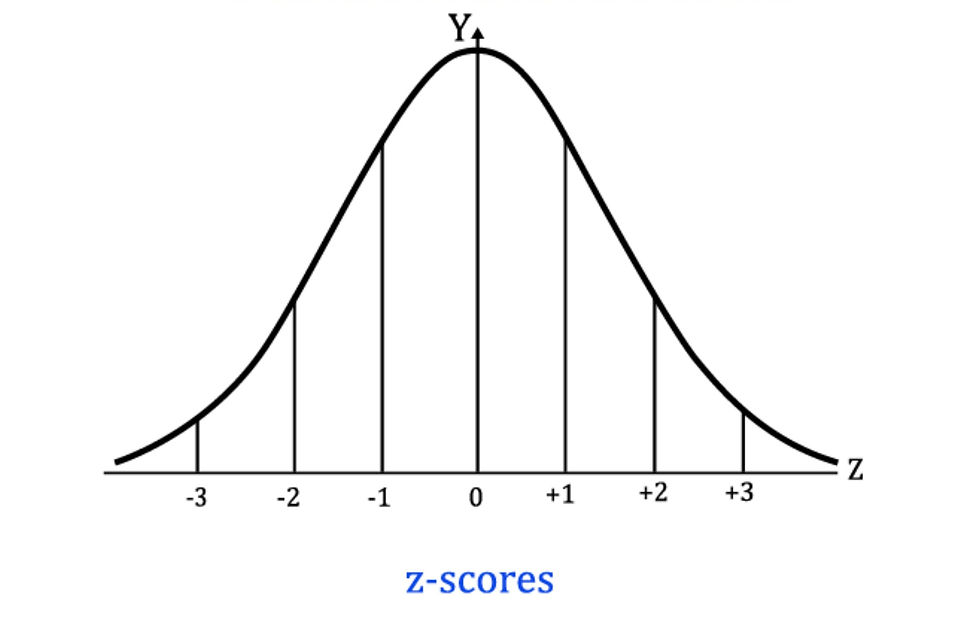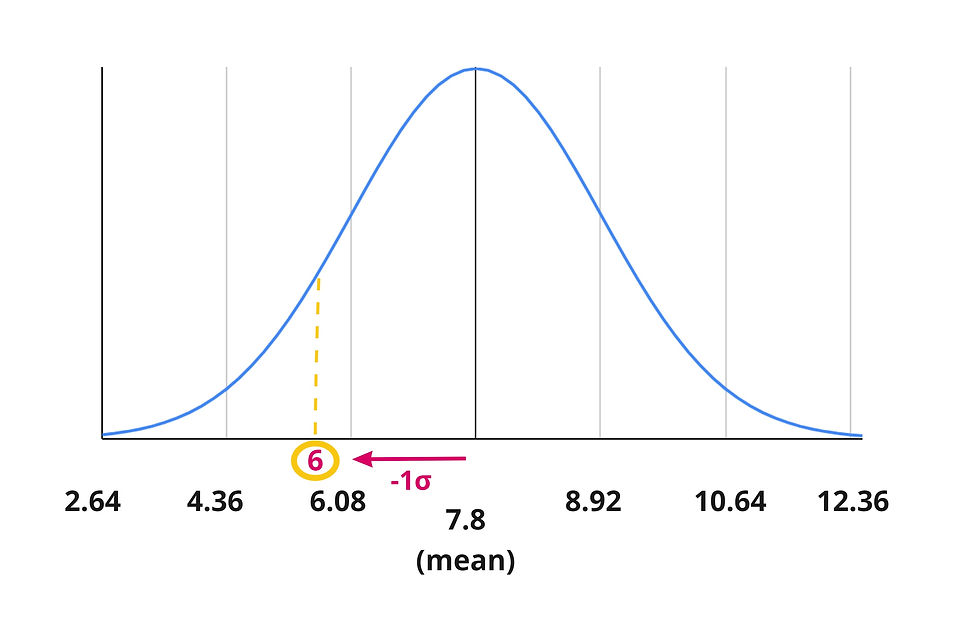top of page

# Z DistributionThe Z table gives us the probability of getting any z-score on a standardized normal bell curve.

Here's an overview about z-scores and how to use the Z-Table.

## What is a Z Score?

A z-score is a standardized value of a data point in our dataset.

The actual value of the data point is "standardized" or adjusted to express how many standard deviations it is from the mean of the dataset.

To convert a value in your dataset to a z-score, you can use the following equation:x = the data point

μ = mean

σ = standard deviation

Subtracting the mean from a data point (x - μ) gives us the distance between the data point and the mean of the data set.

Dividing a point's distance from the mean (x - μ) by the standard deviation (σ) of the dataset tells us how many standard deviations it is away from the mean.

## Probability

We can find out what the chances are of getting a value in a dataset using it's z-score. We can find the probability or p-value of a z-score by either looking at a Z-table or using a calculator.

## Example

##### We can use our mean and standard deviation to find the z-score for the actual value of 6:x = 6 μ = 7.8 σ = 1.72The Z-score for our raw score of 6 is approximately -1.05.

Therefore, a test score of 6 is 1.05 standard deviation below the mean of our data set.Remember - we can find the probability of getting any value in our dataset as long as we know how many standard deviations it is away from the mean (it's z-score):

Here's how:

## Empirical Rule

According to the Empirical Rule,

68% of the data falls within 1 standard deviation of the mean

95% of the data falls within 2 standard deviations of the mean

99.7% of the data falls within 3 standard deviations of the meanTherefore, the bell curve breaks down into the following areas of probability (out of 100%):These distances from the mean can be represented as z-scores:So in our example, our raw score of 6 points:Has a z-score of -1.05:So using our knowledge of the bell curve we can estimate the probability of obtaining a score of 6 or less:Conversely, we can also find the probability of scoring more than 6 using the rules of normal probability:## Z-Table

Rather than estimating, we can get a precise probability value for our z-score of 6 using a Z-Table.

You can look up your z-score in two steps:

* Your z-score should be rounded to two decimal places.

Step 1. Find the value of the first two digits (immediately before and after the decimal point) of your z-score along the left column of the table.

Step 2. Find the corresponding row that contains the last digit (in the hundredths place) of

For example, our we can look up our z-score of -1.05 by finding the -1.0 value in the column of the Z-table, and then finding the row that contains .05.The probability value that we find for our z-score is .1469 or 14.69%.Z-table gives you the area to the left of the Z-score. (The probability of getting a score less than or equal to that value.)(Remember, all of the values inside of the Z table add up to 1 or 100%)

Our estimate of less than 16% using the empirical rule was very close!However, the table's probability value of .1469 or about 15% is exact!

To find the area to the right of the Z-score (the probability of scoring higher more than a given value), you must subtract the probability from one (1 - probability).By subtracting our probability from 1, (1-.1469) we find there is a .8531 or 85.31% probability of scoring greater than 6 on the test.

This is close to our approximation of greater than 84%. (We're on a roll!)## Calculators

The easiest way to calculate a z score, and find it's probability (p-value) is to use a statistics calculator.

Graphing calculators, statistical software programs (such as Excel, SPSS, R), or free online calculators are all able to Z-Tests, which give you the z-score and resulting probability for a value in a dataset.